# PRIMES vs NO-PRIMES

## ABSTRACT

Identifying the PRIMES (P) from the NON-PRIMES or NO-PRIMES (NP) from the pool of ODD numbers is a matter of separation, as one defines the other. Two new methods have been found: the algebraic method and the algebraic-geometry method. Both methods capture ALL the NP and visually come together in the geometry. The focus here is on the algebraic method.

The equation, 6yx +/- y = NP, will identify ALL NP*. Eliminating these NP from the the ODD number pool will leave only the P. A confirmation of primality (but not proof) is found simply be showing the difference in the squares of ANY two P(>3) is a multiple of 24.

*True if ÷3 ODDs are first eliminated, otherwise ADD exponentials of 3 to the NP pool.

Any ODD number can be proved to a a NP if, and only if, its ODD factor (y) divides evenly said ODD/6, as reduced by x, where x=1,2,3,..

A distinct Number Pattern Sequence (NPS) of the NP defines the elusive pattern of the P.

### INTRODUCTION and PREMISE

Identifying the PRIMES (P) from the NON-PRIMES or NO-PRIMES (NP) from the pool of ODD numbers is a matter of separation — as one defines the other. Equation (1) will identify ALL NP.

(1)                  * $6yx ± y = NP$

let y = odd number (ODD) 3, 5, 7,… and x = 1, 2, 3,...

*True if ÷3 ODDs are first eliminated, otherwise ADD exponentials of 3 to the NP pool.

It is advantageous to initially remove ALL ODDs ÷3 — and similarly from the NP÷3 — as this reduces the pool by a third. The remaining NP set = AR, an Active Row, while the ÷3 ODDs = NON-AR set.

A necessary, but not sufficient confirmation — but not proof — of primality is found by finding the even division of 24 into the difference of the square of ANY two PRIMES as:

(2)                  (P2)2 - (P1)2 = $n24$

let $n = 1, 2, 3,...$

Be aware that this also holds true for ALL the AR NP. The P and NP are NOT ÷3, and are both part of the ARS and therefore any combination of the two squared differences will be ÷24:

(3)                  (NP2)2 - (NP1)2 = $n24$

(4)                  (NP2)2 - (P1)2 = $n24$

(5)                  (P2)2 - (NP1)2 = $n24$

The ÷3 NON-AR set is separately ÷24, but can NOT be mixed with members of the AR set (ARS) as:

(6)                  (NON-AR-NP2)2 - (NON-AR-NP1)2 = n24

(7)                  (NON-AR-NP2)2 - (NP1)2$n24$

(8)                  (NP2)2 - (NON-AR-NP1)2$n24$

(9)                  (P2)2 - (NON-AR-NP1)2$n24$

(10)                (NON-AR-NP2)2 - (P1)2$n24$

The division into AR and NON-AR sets has a NPS that ultimately define the elusive pattern of the P.

Furthermore, $6yx ± y = NP$ may be re-arranged to:

(11)                  $(NP ± y)/6yx = 0$

(12)                  $(NP ± y)/6x = y$

asking whether any given ODD (>3) is a P or NP, it is exclusively a NP if, and only if, y reduces to the same value after applying x. As y is effectively an ODD of either a PRIME or composite of PRIMES factor*, one only needs to satisfy a single instance to validate NON-Primality.

If, and only if, $(NP ± y) )/6x = y$ is true, then that ODD number is a NP.

*The various instances includes composites of the given prime factors, but only the pure prime factors need to be satisfied.

### CONCLUSION

The NP form a distinct NPS, and in doing so, they draw out the opposing — and elusive — pattern of the P.

A positive and negative space collectively forming an image shape is apparent.

The philosophical paradox questions which is the positive and which is the negative space.

As in the matter-antimatter duality, our preferred frame of reference limits us from seeing — and likely fully experiencing — the whole.

As ALL NP can be easily surmised via a spreadsheet, eliminating these from the pool of ODDs surveyed, will reveal ALL the P. This may be done for any contiguous run of ODDs. This inclusive-exclusive approach will not only predict all larger P, it will, indeed, reveal the possibility of ALL hidden primes between — as with those between the larger Mersenne Primes.

### BACKGROUND

The full details of the research and discoveries are documented here.

• A relationship between the P and 24 was found in 2005 (link)

• Years later, a right-angle, squared numbers relationship between the ODD number summation series and the Inverse Square Law (ISL) lead to the discovery of the BIM (BBS-ISL Matrix).

• The BIM is infinite and ubiquitous in presenting the known relationship of natural Whole Integer Numbers (WIN) in describing the Universal Law of the ISL. It is easily laid out in a spreadsheet app.

• The Pythagorean Triples — Pythagorean Triangles composed exclusively of WIN — are also infinitely and ubiquitously embedded within the BIM. There is a connection of the Primitive PTs (PPTs) to the P.

• TPISC I, II, III, IV (The Pythagorean–Inverse Square Connection) fully describes the Basic, Advanced, Clarity and the Tree of Pythogorean Triples, and Details of the PPTs and PRIMES, as well as the Double-Slit Experiment and Quantum Entanglement Conjecture (DSEQEC), as part of the larger Creation and Conservation of SpaceTime Conjecture (CaCoST).

• Dividing the WIN within the BIM by 24 — BIM÷24 — has led to the discovery:

• ALL PPTs and ALL PRIMES satisfy a necessary, but not sufficient, condition that they ONLY fall on NOT ÷3 ODD numbered Rows on the BIM;

• Such Row are referred to as Active Rows (ARs) within an ODD–EVEN–ODD Row set, the Active Row Set (ARS);

• ALL ÷3 Row — NON-ARs — alternate with the ARs on the BIM;

• The NPS of ALL NP:

• those ÷3 = NON-AR
• those NOT ÷3 = AR

reveal ALL NP and in doing so, by elimination, reveal ALL P. See supporting graphics and tables below;

• Eliminating ALL ODDs ÷3 removes ALL NP÷3, simplifying the base of ODDs to ONLY those on ARS;

• $6yx ± y = NP$ identifies ALL NP*;

*True if ÷3 ODDs are first eliminated, otherwise ADD exponentials of 3 to the NP pool.;

• PRIMES (P) are ALL that remain once the NP are eliminated;

• $n24$ divides EVENLY into:

• ALL differences between ANY two squared ARs — includes both P and NOT÷3 NP;
• ALL differences between ANY two squared NON-ARs — includes exclusively the ÷3NP;
• NO cross-over between the two sets allowed.
• Re-arranging $6yx ± y = NP$ , one may ask if any ODD number is P or NP;

• ODD = NP if, and only if, the y factor reduces to the same value upon dividing the ODD ±y by 6x;
• ODD = PRIME if, and only if, no y factor reduces to the same value.

## SUMMARY*

### How do we go from the simple grid of the BIM (BBS-ISL Matrix) to identifying the PRIMES?

1. The BIM is a symmetrical grid — divided equally down its diagonal center with the Prime Diagonal (PD) — that illuminates the Number Pattern Sequence (NPS) of the Inverse Square Law (ISL) via simple, natural Whole Integer Numbers (WIN).

2. The BIM Axis numbers are 1,2,3,.. with 0 at the origin.

3. The Inner Grid (IG) contains EVEN and ODD WIN, but except for the 1st diagonal next to the PD — a diagonal that contains ALL the ODD WIN — there are NO PRIMES (NO-PRIMES, NP) on the SIG (Strict Inner Grid).

4. The PD WIN are simple the square of the Axis WIN.

5. ALL the IG WIN result from subtracting the horizontal from the vertical intersection of the PD.

6. Dropping down a given PD Squared WIN (>4) until it intersects with another squared WIN on a Row below will ALWAYS reveal that Row to be a Primitive Pythagorean Triple (PPT) Row, whose hypotenuse, c, lies on the intersecting PD. ALL PPTs may be identified this way.

7. Dividing the BIM cell values by 24 — BIM÷24 — forms a criss-crossing DIAMOND NPS that divides the overall BIM into two distinct and alternating Row (and Column) bands or sets:

• ODD WIN that are ÷3 and referred to as NON-ARs;
• ODD WIN that are NOT ÷3 and referred to as ARs, or Active Rows;
• The ARs ALWAYS come in pairs — with an EVEN WIN between — as the UPPER and LOWER AR of the ARS (Active Row Set);
8. ALL PPTs and ALL PRIMES ALWAYS are found exclusively on the ARs — no exceptions.

9. By applying:

(1)                  * $6yx ± y = NP$

let y = odd number (ODD) 3, 5, 7,… and x = 1, 2, 3,... one generates a NP table containing ALL the NP;

*True if ÷3 ODDs are first eliminated, otherwise ADD exponentials of 3 to the NP pool;

10. Eliminating the NP — and the NP contain a NPS — from ALL the ODD WIN, reveals the PRIMES (P).

11. A necessary, but not sufficient confirmation — but not proof — of primality is found by finding the even division of 24 into the difference of the square of ANY two PRIMES as:

(2)                  (P2)2 - (P1)2 = $n24$

let $n = 1, 2, 3,...$

Be aware that this also holds true for ALL the AR NP. The P and NP are NOT ÷3, and are both part of the ARS and therefore any combination of the two squared differences will be ÷24:

(3)                  (NP2)2 - (NP1)2 = $n24$

(4)                  (NP2)2 - (P1)2 = $n24$

(5)                  (P2)2 - (NP1)2 = $n24$

The ÷3 NON-AR set is separately ÷24, but can NOT be mixed with members of the AR set (ARS) as:

(6)                  (NON-AR-NP2)2 - (NON-AR-NP1)2 = n24

(7)                  (NON-AR-NP2)2 - (NP1)2$n24$

(8)                  (NP2)2 - (NON-AR-NP1)2$n24$

(9)                  (P2)2 - (NON-AR-NP1)2$n24$

(10)                (NON-AR-NP2)2 - (P1)2$n24$

The division into AR and NON-AR sets has a NPS that ultimately define the elusive pattern of the P.

Furthermore, $6yx ± y = NP$ may be re-arranged to:

(11)                  $(NP ± y)/6yx = 0$

(12)                  $(NP ± y)/6x = y$

asking whether any given ODD (>3) is a P or NP, it is exclusively a NP if, and only if, y reduces to the same value after applying x. As y is effectively an ODD of either a PRIME or composite of PRIMES factor*, one only needs to satisfy a single instance to validate NON-Primality.

12. One can also obtain ALL the P by eliminating the BIM SIGO and O2 from the 1st Diagonal WIN, where SIGO = Strict Inner Grid ODDs, O2 = ODDs2, and the 1st Diagonal = the 1st Diagonal Parallel to the PD.

This itself is further simplified by switching out the ODD AXIS values with the O2 — the O2 being the PD values — such that we now have:

(13)   SIGO(A2) = Strict Inner Grid ODDS & ODD AXIS2 giving a distinct visualization advantage;

(14)                  NP = SIGO(A2)

(15)                  1st Diagonal - SIGO(A2) = P.

This second method — the algebraic geometry method — is presented here. (Simple Path from the BIM to the PRIMES.)

### SUPPORTING GRAPHICS AND TABLES

NOTE: As each PDF contains several or more tables, OPEN them separately in a separate TAB/WINDOW.Table31a5_+NO-PRIME_EQUATION_Pattern_Factors. Starts with the BIM ARS, finishes with a large table demo that shows *NP=6yx +/- y and y= (NP +/- y)/6x. Layout and explanatory note at the bottom.

*True if ÷3 ODDs are first eliminated, otherwise ADD exponentials of 3 to the NP pool.;

NOTE: The NP tables have TWO MAJOR Columns designated "LOWER" and "UPPER." Each MAJOR Column has TWO parts: solving for NP=6yx +/- y is LEFT, solving for y= (NP +/- y)/6x is RIGHT.

For any ARS (coming from the BIM), the "LOWER" refers to the Left MAJOR Column on any of the NP tables and the "UPPER" refers to the Right Column. The difference is in the +/- sign action for the LEFT side of each MAJOR Column: if y is subtracted (-), it is on the "LOWER" number of the ARS on the BIM, if y is added (+), it is on the "UPPER" number of that ARS set.

When solving for y= (NP +/- y)/6x on the RIGHT of each MAJOR Column, the +/- signs are reversed!

At first, this may seem confusing, but is really just a consistent pattern of the mathematics and is easily seen once you see how the BIM÷24 generates the ARS that leads to the NP Equation Tables that in turn reveal the Number Pattern Sequences (NPS) that reveal the pattern of the PRIMES (P).

The PDFs will follow these animated gifs. Videos and other supporting graphics thereafter.

Animated Gifs: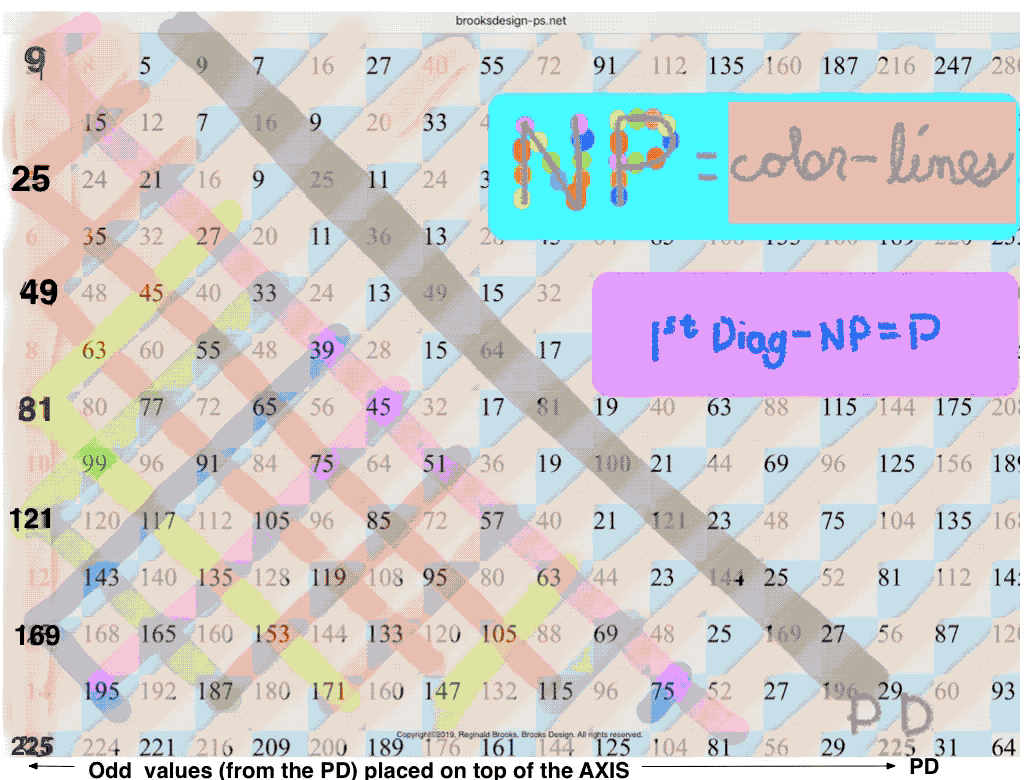PRIMES vs NO-PRIMES-2: algebraic method.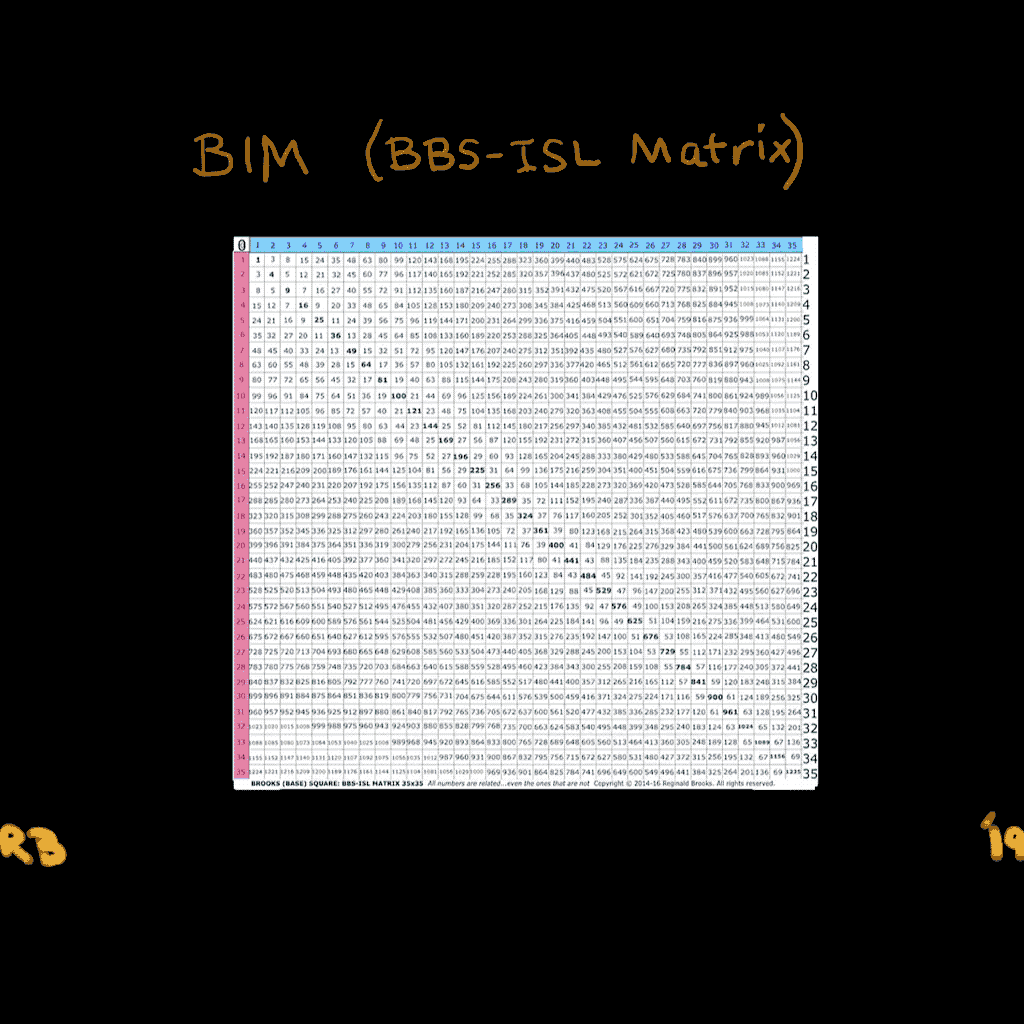PRIMES vs NO-PRIMES-1: algebraic-geometry method.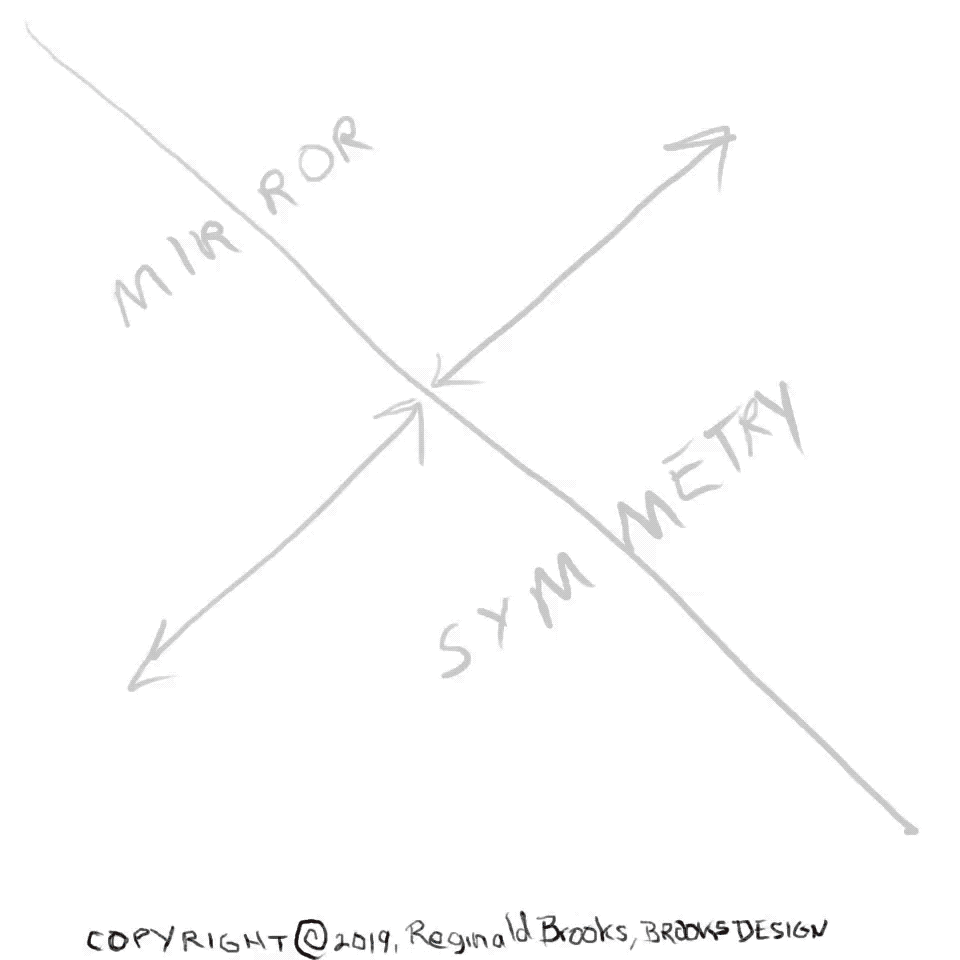PRIMES vs NO-PRIMES-3: algebraic and algebraic geometry method.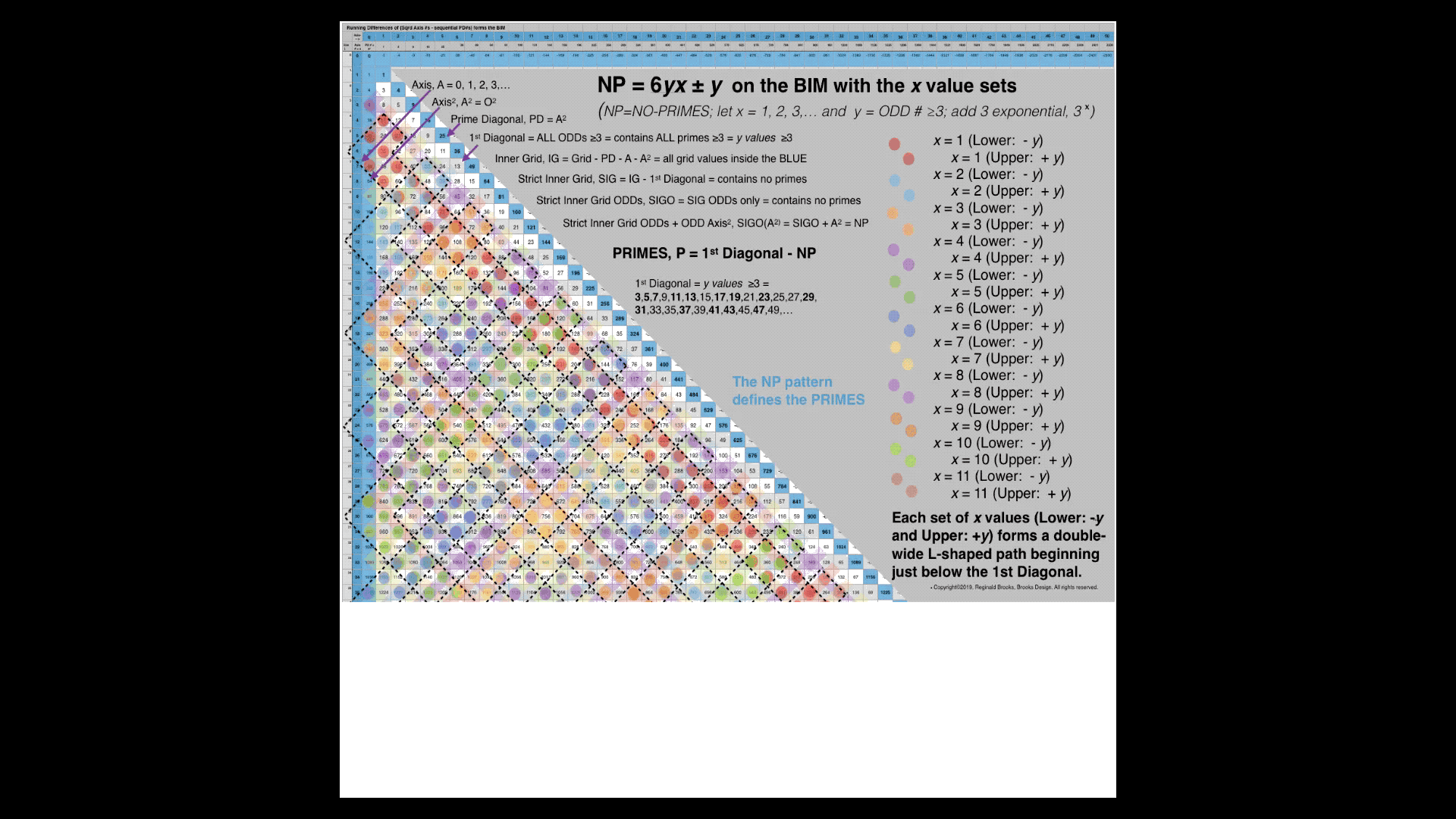PRIMES vs NO-PRIMES-4: algebraic method in detail.

PDFs +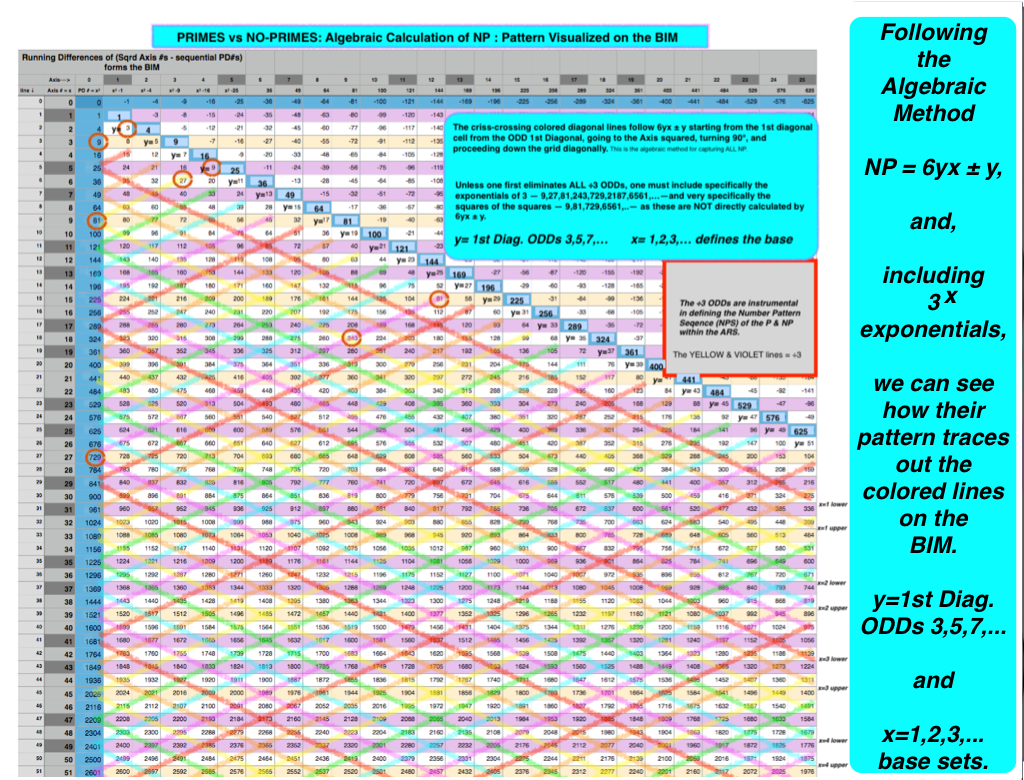PRIMES vs NO-PRIMES: snapshot-1 showing dovetailing visualization of the algebraic method of calculating the NP with that of the geometric method on the BIM. The larger PDF is below.PRIMES vs NO-PRIMES PDF: snapshot-1 showing dovetailing visualization of the algebraic method of calculating the NP with that of the geometric method on the BIM. The NPS of the NP define the elusive pattern of the P.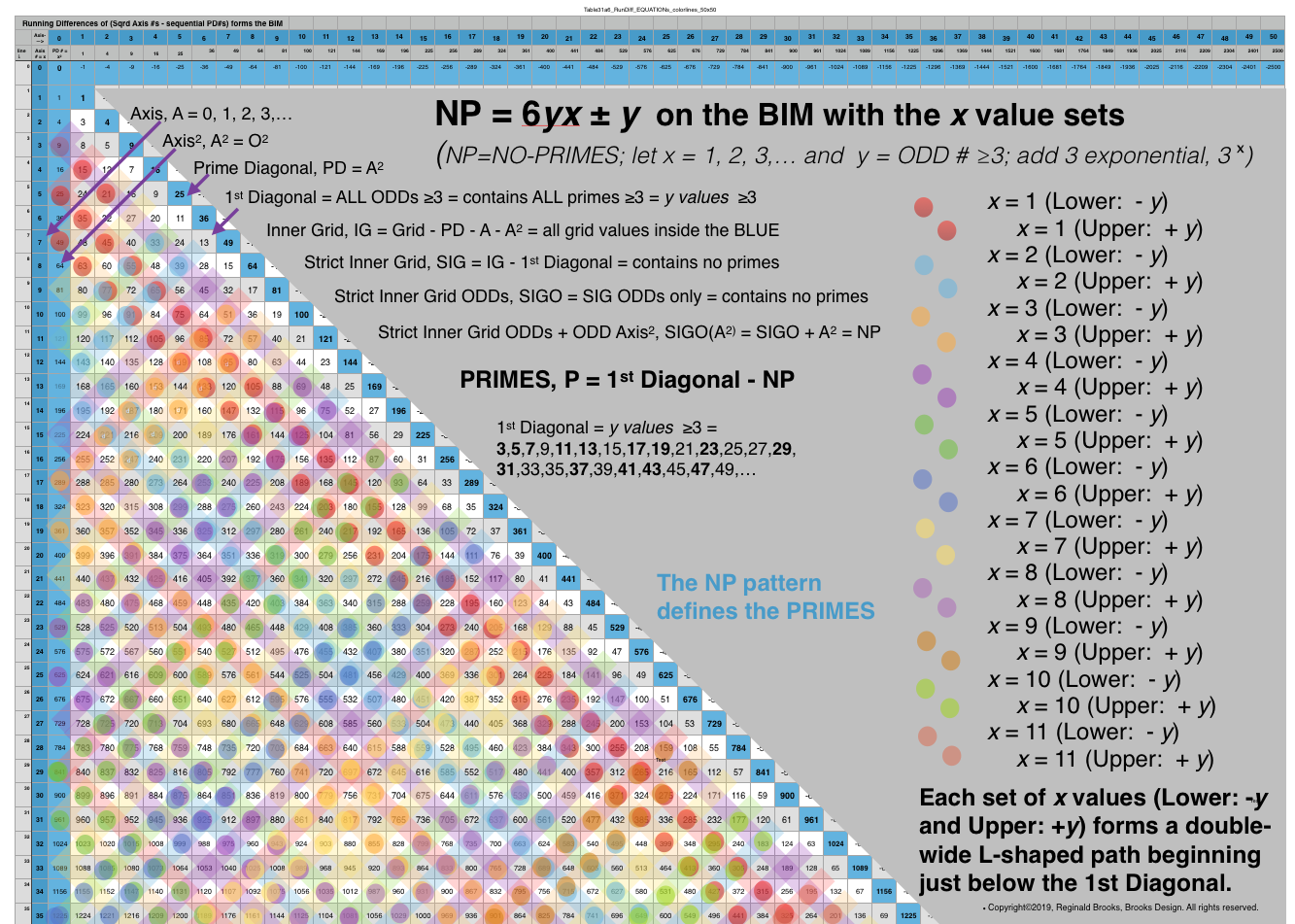PRIMES vs NO-PRIMES: snapshot-2 showing dovetailing visualization of the algebraic method of calculating the NP with that of the geometric method on the BIM. Here the x=1,2,3,... base value sets -- Lower -y an Upper +y--as double-wide, L-shaped paths beginning just below the 1st Diagonal. These double rows alternate with divisible by 3--or divisible by 9 Axis squared--paths. The larger PDF is below.PRIMES vs NO-PRIMES PDF: snapshot-2 showing dovetailing visualization of the algebraic method of calculating the NP with that of the geometric method on the BIM. The larger PDF is below. Here the x=1,2,3,... base value sets -- Lower -y an Upper +y--as double-wide, L-shaped paths beginning just below the 1st Diagonal. These double rows alternate with divisible by 3--or divisible by 9 Axis squared--paths.PRIMES vs NO-PRIMES: snapshot-3 showing dovetailing visualization of the algebraic method of calculating the NP with that of the geometric method on the BIM. Here the x=1,2,3,... base value sets -- Lower -y an Upper +y--as double-wide, L-shaped paths beginning just below the 1st Diagonal. These double rows alternate with divisible by 3--or divisible by 9 Axis squared--paths. The larger PDF is below.PRIMES vs NO-PRIMES PDF: snapshot-3 showing dovetailing visualization of the algebraic method of calculating the NP with that of the geometric method on the BIM. The larger PDF is below. Here the x=1,2,3,... base value sets -- Lower -y an Upper +y--as double-wide, L-shaped paths beginning just below the 1st Diagonal. These double rows alternate with divisible by 3--or divisible by 9 Axis squared--paths.PRIMES vs NO-PRIMES PDF: snapshot-4 showing dovetailing visualization of the algebraic method of calculating the NP with that of the geometric method on the BIM. The larger PDF is below. Here the x=1,2,3,... base value sets -- Lower -y an Upper +y--as double-wide, L-shaped paths beginning just below the 1st Diagonal. These double rows alternate with divisible by 3--or divisible by 9 Axis squared--paths.Table31a4: PRIMES vs NO-PRIMES PDF: Open in a separate tab/window to see all 11 pages. Here the x=1,2,3,... base value sets -- Lower -y an Upper +y--are shown individually and collectively as sets. The full Upper and Lower tables conclude.Table31a6_2: PRIMES vs NO-PRIMES PDF: If you look at the ODD Axis ÷3 NO-PRIMES (NP) that lie in the paths between the L-shaped Double-wide x-base sets (x=1,2,3,)…), one finds a distinct Number Pattern Sequence (NPS) between successive NP values. Those shown in BLUE are NOT included in the criss-crossing L-shaped Double-wide paths (seen in the snapshots above), while those in GRAY are. The NPS seen here, based ultimately on the 1,3,5,7,… ODD number summation series that defines the whole BIM distribution (including the 1st Diagonal, the PD, and the successive differences in sequential Inner Grid cell values) reiterates that of both the L-shaped Double-wide paths as well as the individual x paths. Both give a NPS of the NP that reveal the elusive pattern of the PRIMES. There remains little doubt that the PRIMES , as well as the Primitive Pythagorean Triples (PPTs), are intimately related to the INVERSE SQUARE LAW (ISL)!

Videos:

...more supporting graphics and tables:Table31a4-5_+RunDiff_EQUATIONsDEMO(÷3Filter)+10x10+. A simple table to demo the NP process.Table31a4_+RunDiff_EQUATION-PATTERNS-LOWER+UPPER+Annotated. NP Table with layout notes.Table31a4_+RunDiff_EQUATIONs(SqrdAxis-seqPD)+50x500+.pdf. From the BIM to the NP/P Tables.BIM35-Table31a4-5_NO-PRIMES_Factores-withARS-YELLOW-numbAnnotated. The NP pattern on the BIM.Table33a,c,d_ODDsqrd÷24_sheets. The NP pattern – an amazing NPS – defines the P.BIMrows1-1000+Primes_sheets+Sub-Matrix2. A BIM reference showing ÷24 ARS, PPTs, NP an P.

For the full documentation of all the graphics and pdfs — including history, development, expanded tables, and more — see the link references immediately below.

### REFERENCES

While there are no specific references for this work other than referring back to my own original work, there are many references involved in the study and research on the PRIMES in general. These have been well documented in the TPISC_IV: Details_BIM+PPT+PRIMES focus white paper. This paper also contains the background graphics and tables leading up to this current work. The focus page is part of the much larger TPISC_IV: Details ebook project that is freely available as an HTML webpage. The quick reference outline can be found here.

~~ ~~ ~~ ~~ ~~ ~~ ~~ ~~ ~~ ~~ ~~ ~~ ~~ ~~ ~~ ~~ ~~

# TPISC_IV:_Details ~PRIMES vs NO-PRIMES~